# Frank Solutions for Class 9 Maths Chapter 2 Profit, Loss and Discount

Frank Solutions for Class 9 Maths Chapter 2 Profit, Loss and Discount provide students with a clear knowledge of the important concepts discussed in the chapter. The solutions are helpful in enhancing problem-solving and logical thinking abilities among students. They are suggested to practise these solutions on a daily basis to boost their exam preparation. Students can refer to the Frank Solutions for Class 9 Maths Chapter 2 Profit, Loss and Discount PDF, which is provided in the link below.

Chapter 2 has important concepts of profit, loss and discount, and the steps used in determining them are explained in brief in this chapter. The solutions are provided in a descriptive manner to make learning interesting for the students. After working on chapter-wise problems from the textbook, they can follow these solutions to understand the various methods which can be used to solve the problems quickly.

## Frank Solutions for Class 9 Maths Chapter 2 Profit, Loss and Discount – Download the PDF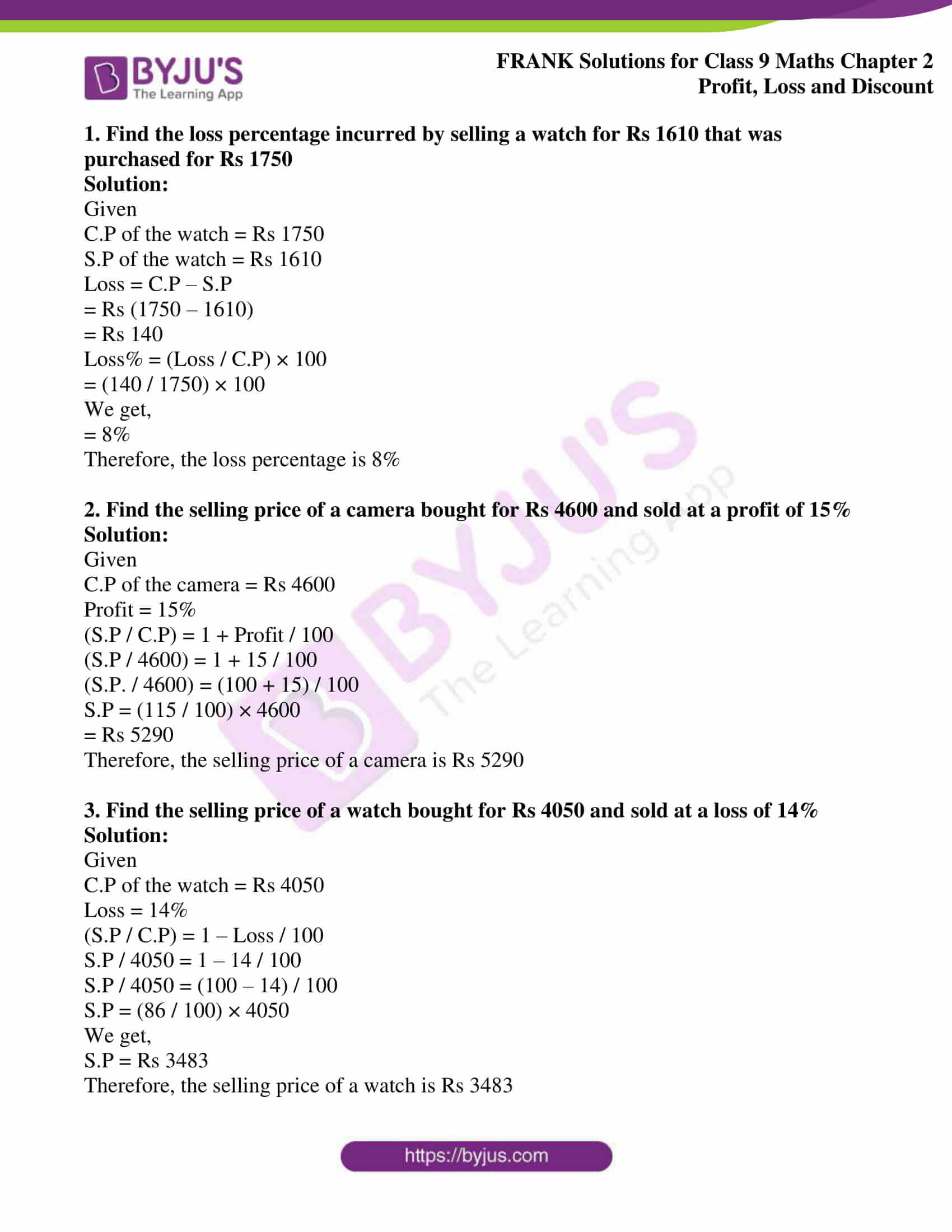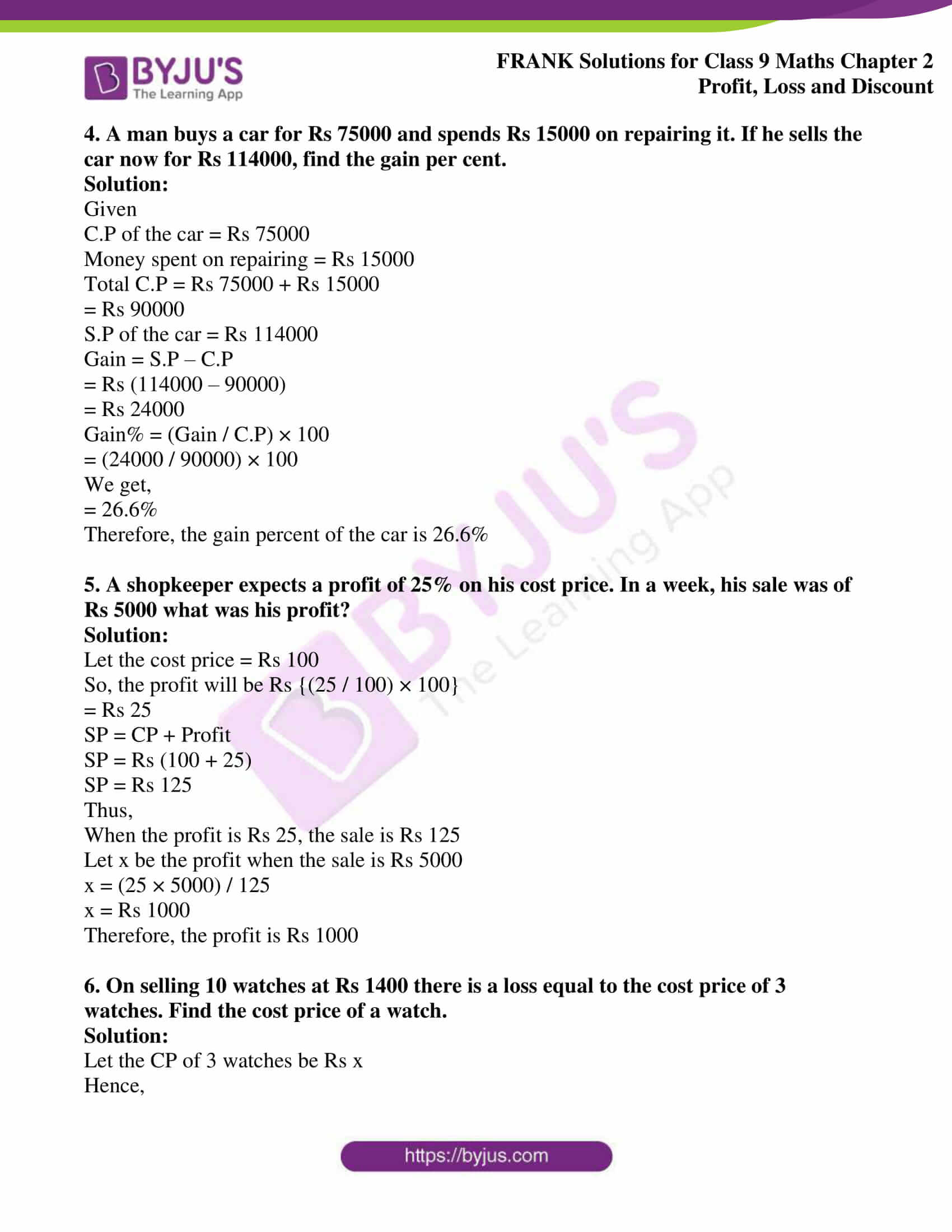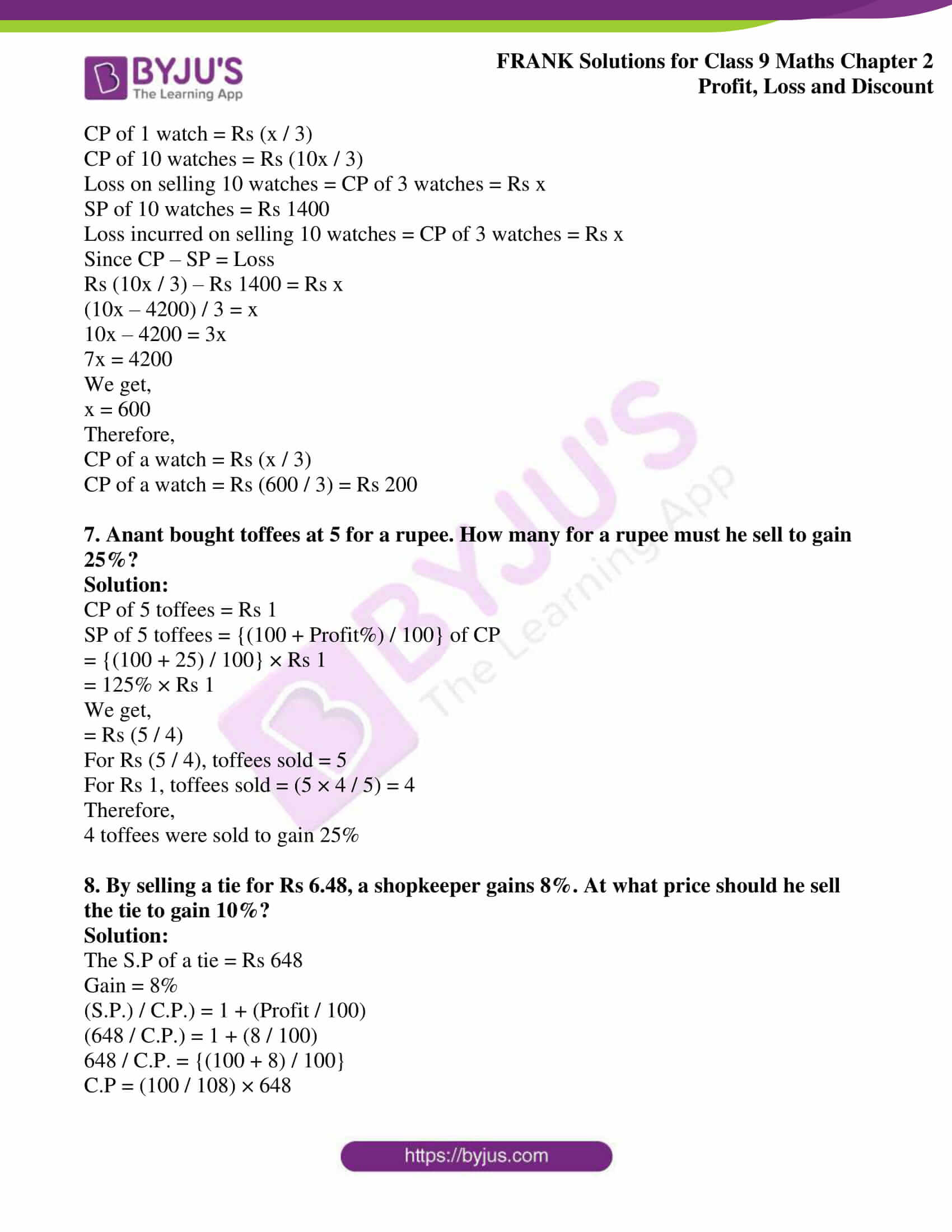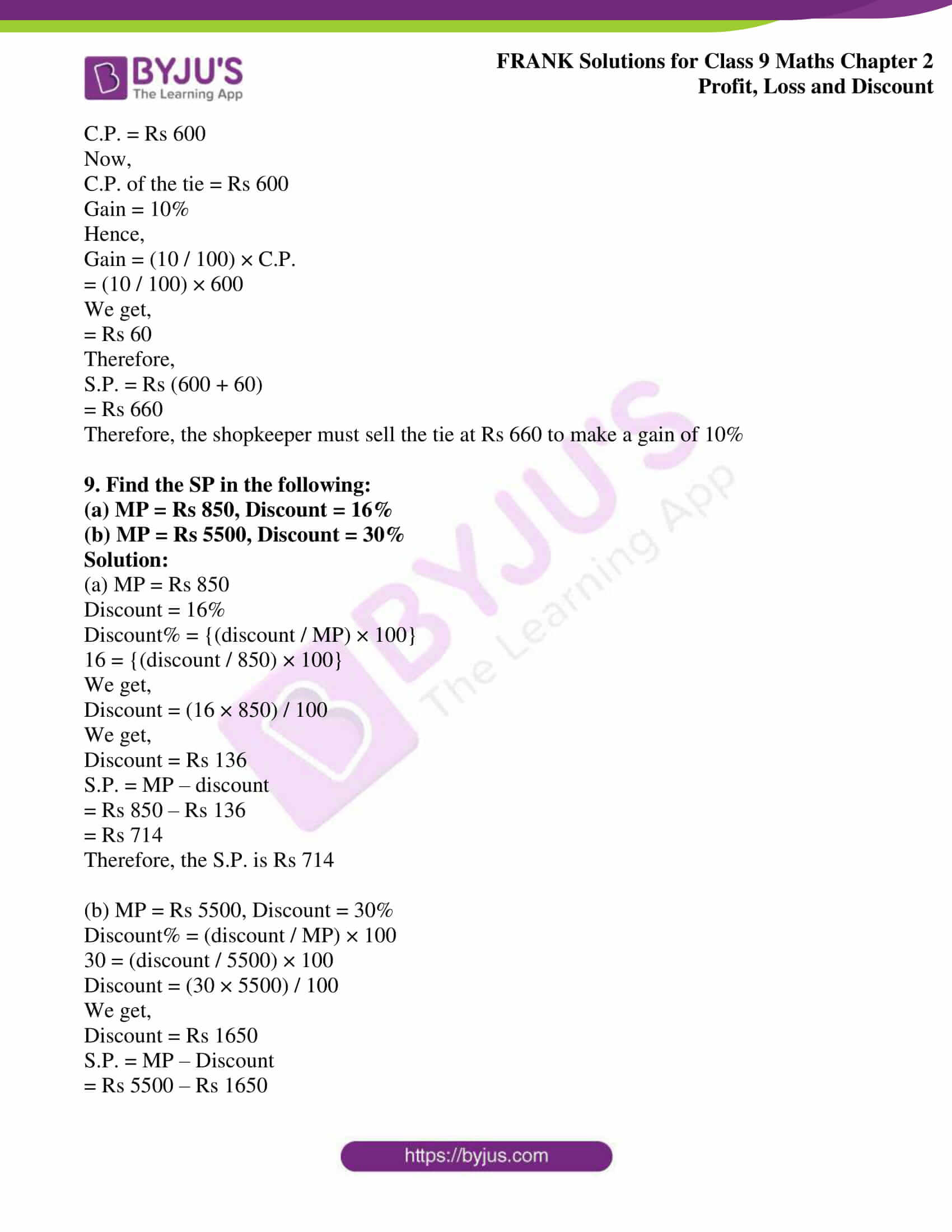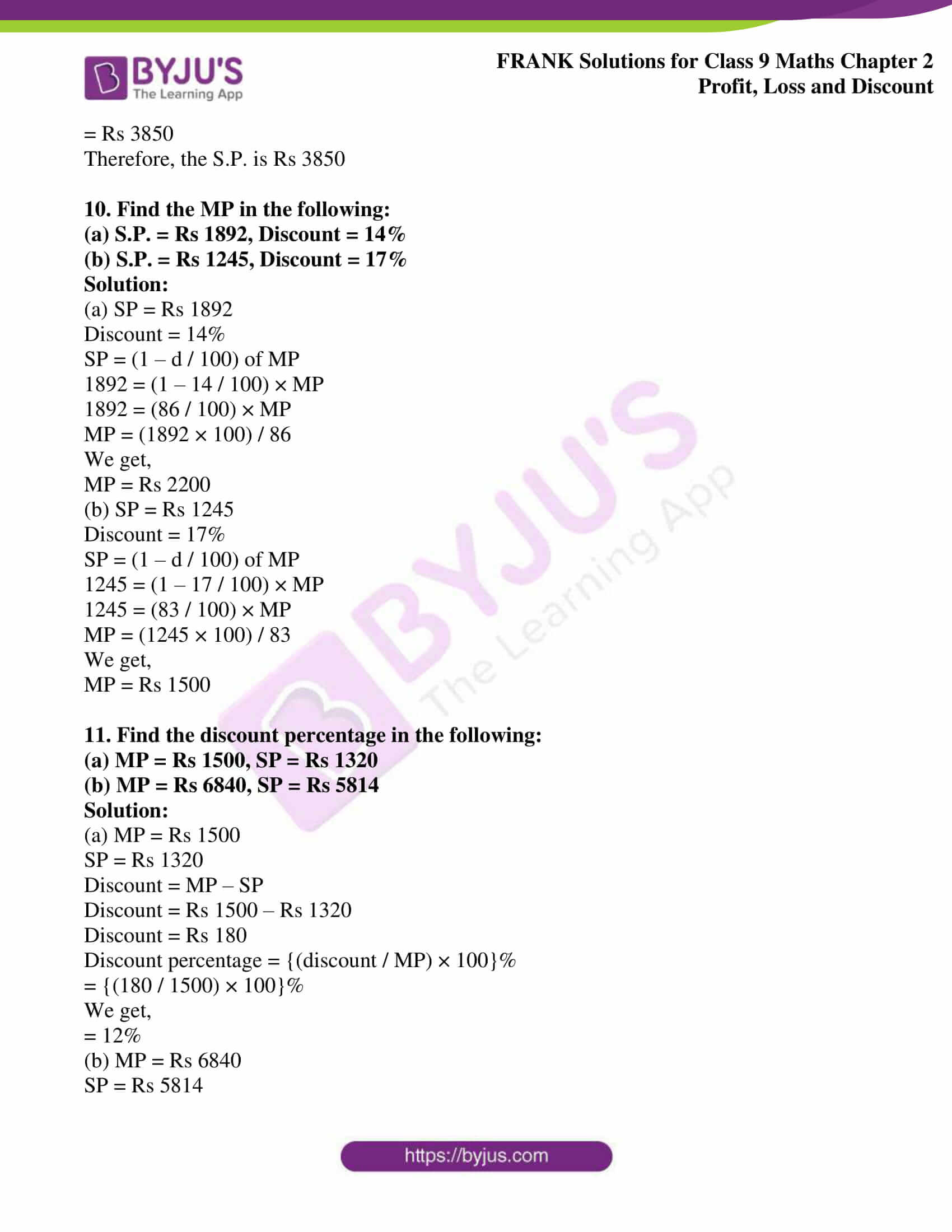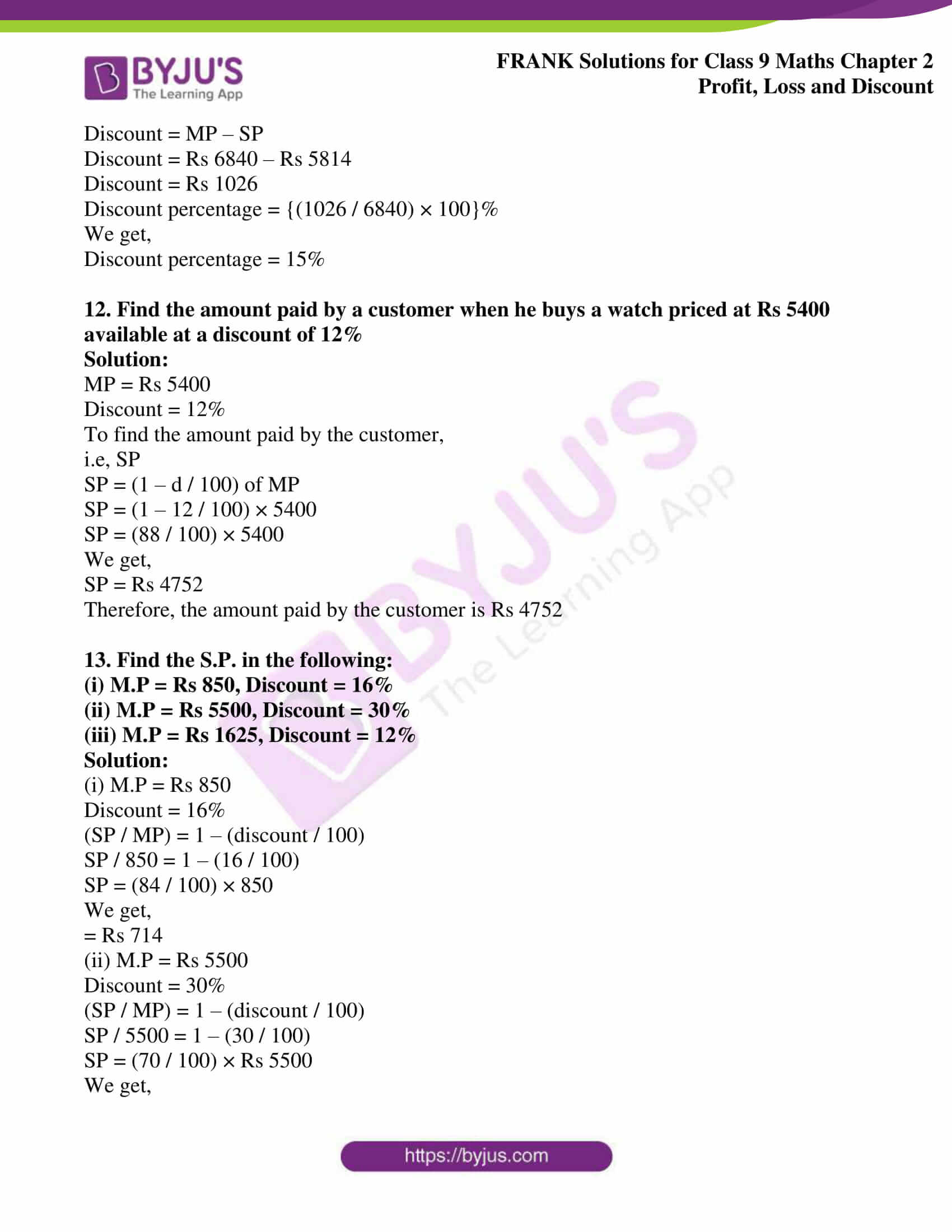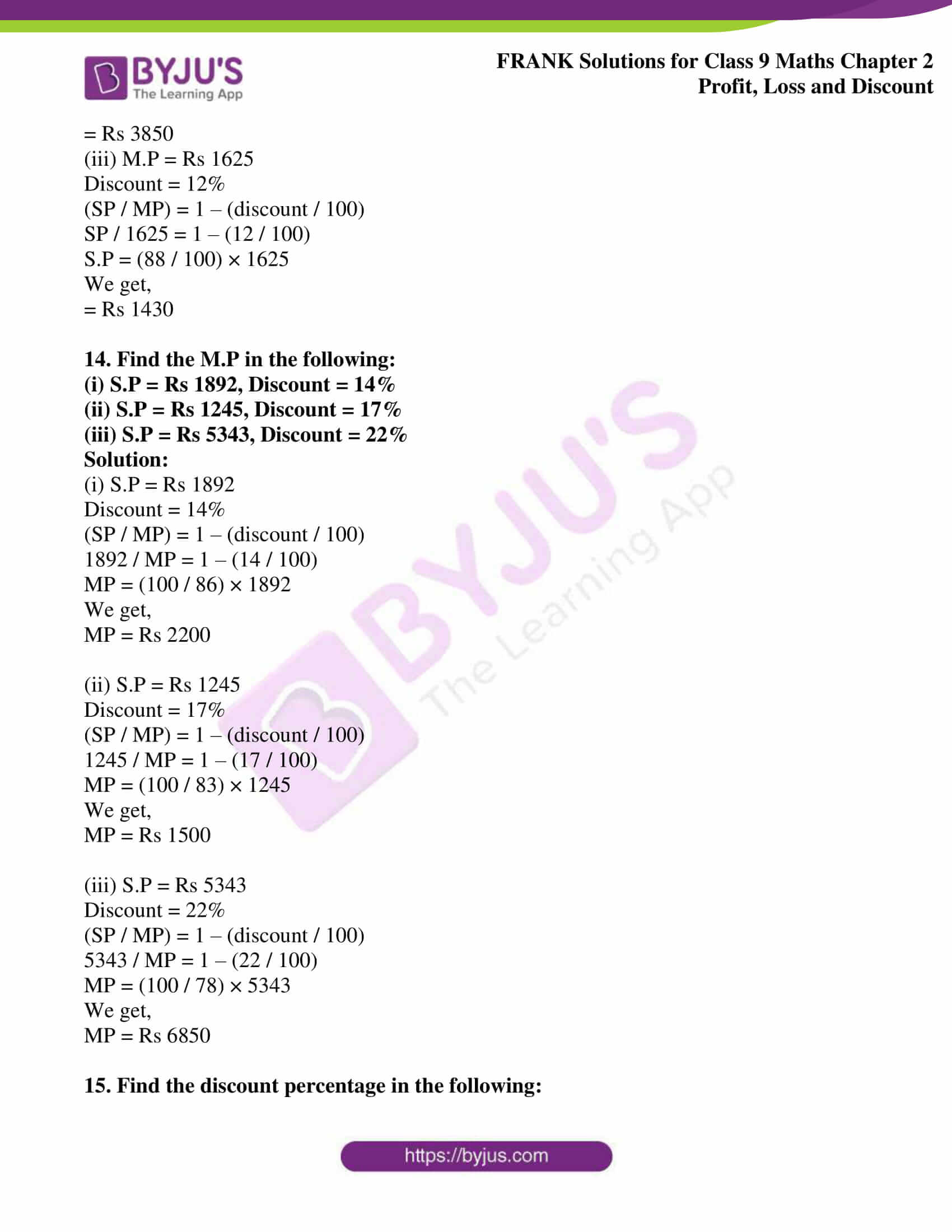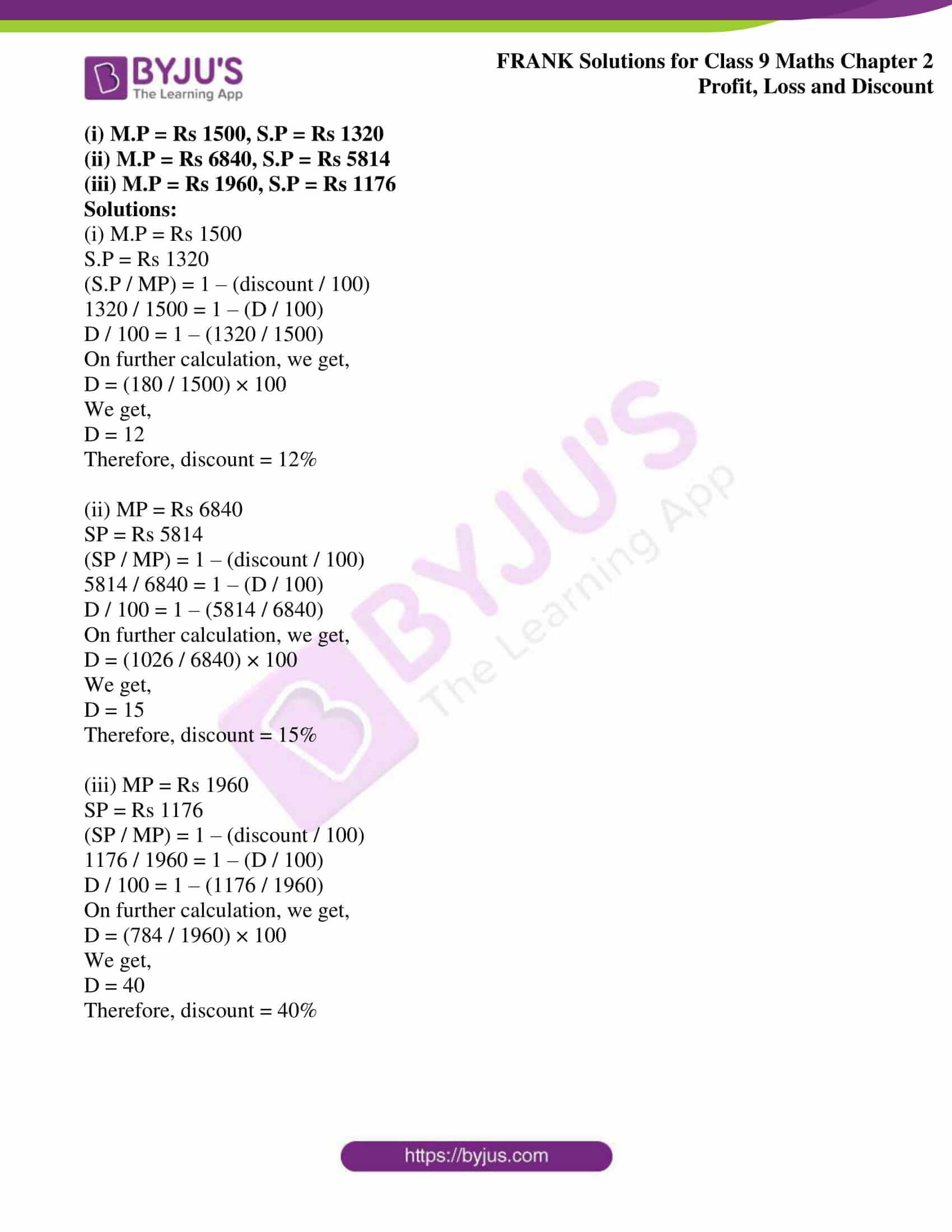## Access Frank Solutions for Class 9 Maths Chapter 2 Profit, Loss and Discount

1. Find the loss percentage incurred by selling a watch for Rs 1610 that was purchased for Rs 1750

Solution:

Given

C.P of the watch = Rs 1750

S.P of the watch = Rs 1610

Loss = C.P – S.P

= Rs (1750 – 1610)

= Rs 140

Loss% = (Loss / C.P) × 100

= (140 / 1750) × 100

We get,

= 8%

Therefore, the loss percentage is 8%

2. Find the selling price of a camera bought for Rs 4600 and sold at a profit of 15%

Solution:

Given

C.P of the camera = Rs 4600

Profit = 15%

(S.P / C.P) = 1 + Profit / 100

(S.P / 4600) = 1 + 15 / 100

(S.P. / 4600) = (100 + 15) / 100

S.P = (115 / 100) × 4600

= Rs 5290

Therefore, the selling price of a camera is Rs 5290

3. Find the selling price of a watch bought for Rs 4050 and sold at a loss of 14%

Solution:

Given

C.P of the watch = Rs 4050

Loss = 14%

(S.P / C.P) = 1 – Loss / 100

S.P / 4050 = 1 – 14 / 100

S.P / 4050 = (100 – 14) / 100

S.P = (86 / 100) × 4050

We get,

S.P = Rs 3483

Therefore, the selling price of a watch is Rs 3483

4. A man buys a car for Rs 75000 and spends Rs 15000 on repairing it. If he sells the car now for Rs 114000, find the gain per cent.

Solution:

Given

C.P of the car = Rs 75000

Money spent on repairing = Rs 15000

Total C.P = Rs 75000 + Rs 15000

= Rs 90000

S.P of the car = Rs 114000

Gain = S.P – C.P

= Rs (114000 – 90000)

= Rs 24000

Gain% = (Gain / C.P) × 100

= (24000 / 90000) × 100

We get,

= 26.6%

Therefore, the gain percent of the car is 26.6%

5. A shopkeeper expects a profit of 25% on his cost price. In a week, his sale was of Rs 5000 what was his profit?

Solution:

Let the cost price = Rs 100

So, the profit will be Rs {(25 / 100) × 100}

= Rs 25

SP = CP + Profit

SP = Rs (100 + 25)

SP = Rs 125

Thus,

When the profit is Rs 25, the sale is Rs 125

Let x be the profit when the sale is Rs 5000

x = (25 × 5000) / 125

x = Rs 1000

Therefore, the profit is Rs 1000

6. On selling 10 watches at Rs 1400, there is a loss equal to the cost price of 3 watches. Find the cost price of a watch.

Solution:

Let the CP of 3 watches be Rs x

Hence,

CP of 1 watch = Rs (x / 3)

CP of 10 watches = Rs (10x / 3)

Loss on selling 10 watches = CP of 3 watches = Rs x

SP of 10 watches = Rs 1400

Loss incurred on selling 10 watches = CP of 3 watches = Rs x

Since CP – SP = Loss

Rs (10x / 3) – Rs 1400 = Rs x

(10x – 4200) / 3 = x

10x – 4200 = 3x

7x = 4200

We get,

x = 600

Therefore,

CP of a watch = Rs (x / 3)

CP of a watch = Rs (600 / 3) = Rs 200

7. Anant bought toffees at 5 for a rupee. How many for a rupee must he sell to gain 25%?

Solution:

CP of 5 toffees = Rs 1

SP of 5 toffees = {(100 + Profit%) / 100} of CP

= {(100 + 25) / 100} × Rs 1

= 125% × Rs 1

We get,

= Rs (5 / 4)

For Rs (5 / 4), toffees sold = 5

For Rs 1, toffees sold = (5 × 4 / 5) = 4

Therefore,

4 toffees were sold to gain 25%

8. By selling a tie for Rs 6.48, a shopkeeper gains 8%. At what price should he sell the tie to gain 10%?

Solution:

The S.P of a tie = Rs 648

Gain = 8%

(S.P.) / C.P.) = 1 + (Profit / 100)

(648 / C.P.) = 1 + (8 / 100)

648 / C.P. = {(100 + 8) / 100}

C.P = (100 / 108) × 648

C.P. = Rs 600

Now,

C.P. of the tie = Rs 600

Gain = 10%

Hence,

Gain = (10 / 100) × C.P.

= (10 / 100) × 600

We get,

= Rs 60

Therefore,

S.P. = Rs (600 + 60)

= Rs 660

Therefore, the shopkeeper must sell the tie at Rs 660 to make a gain of 10%

9. Find the SP in the following:

(a) MP = Rs 850, Discount = 16%

(b) MP = Rs 5500, Discount = 30%

Solution:

(a) MP = Rs 850

Discount = 16%

Discount% = {(discount / MP) × 100}

16 = {(discount / 850) × 100}

We get,

Discount = (16 × 850) / 100

We get,

Discount = Rs 136

S.P. = MP – discount

= Rs 850 – Rs 136

= Rs 714

Therefore, the S.P. is Rs 714

(b) MP = Rs 5500, Discount = 30%

Discount% = (discount / MP) × 100

30 = (discount / 5500) × 100

Discount = (30 × 5500) / 100

We get,

Discount = Rs 1650

S.P. = MP – Discount

= Rs 5500 – Rs 1650

= Rs 3850

Therefore, the S.P. is Rs 3850

10. Find the MP in the following:

(a) S.P. = Rs 1892, Discount = 14%

(b) S.P. = Rs 1245, Discount = 17%

Solution:

(a) SP = Rs 1892

Discount = 14%

SP = (1 – d / 100) of MP

1892 = (1 – 14 / 100) × MP

1892 = (86 / 100) × MP

MP = (1892 × 100) / 86

We get,

MP = Rs 2200

(b) SP = Rs 1245

Discount = 17%

SP = (1 – d / 100) of MP

1245 = (1 – 17 / 100) × MP

1245 = (83 / 100) × MP

MP = (1245 × 100) / 83

We get,

MP = Rs 1500

11. Find the discount percentage in the following:

(a) MP = Rs 1500, SP = Rs 1320

(b) MP = Rs 6840, SP = Rs 5814

Solution:

(a) MP = Rs 1500

SP = Rs 1320

Discount = MP – SP

Discount = Rs 1500 – Rs 1320

Discount = Rs 180

Discount percentage = {(discount / MP) × 100}%

= {(180 / 1500) × 100}%

We get,

= 12%

(b) MP = Rs 6840

SP = Rs 5814

Discount = MP – SP

Discount = Rs 6840 – Rs 5814

Discount = Rs 1026

Discount percentage = {(1026 / 6840) × 100}%

We get,

Discount percentage = 15%

12. Find the amount paid by a customer when he buys a watch priced at Rs 5400, available at a discount of 12%.

Solution:

MP = Rs 5400

Discount = 12%

To find the amount paid by the customer,

i.e, SP

SP = (1 – d / 100) of MP

SP = (1 – 12 / 100) × 5400

SP = (88 / 100) × 5400

We get,

SP = Rs 4752

Therefore, the amount paid by the customer is Rs 4752

13. Find the S.P. in the following:

(i) M.P = Rs 850, Discount = 16%

(ii) M.P = Rs 5500, Discount = 30%

(iii) M.P = Rs 1625, Discount = 12%

Solution:

(i) M.P = Rs 850

Discount = 16%

(SP / MP) = 1 – (discount / 100)

SP / 850 = 1 – (16 / 100)

SP = (84 / 100) × 850

We get,

= Rs 714

(ii) M.P = Rs 5500

Discount = 30%

(SP / MP) = 1 – (discount / 100)

SP / 5500 = 1 – (30 / 100)

SP = (70 / 100) × Rs 5500

We get,

= Rs 3850

(iii) M.P = Rs 1625

Discount = 12%

(SP / MP) = 1 – (discount / 100)

SP / 1625 = 1 – (12 / 100)

S.P = (88 / 100) × 1625

We get,

= Rs 1430

14. Find the M.P in the following:

(i) S.P = Rs 1892, Discount = 14%

(ii) S.P = Rs 1245, Discount = 17%

(iii) S.P = Rs 5343, Discount = 22%

Solution:

(i) S.P = Rs 1892

Discount = 14%

(SP / MP) = 1 – (discount / 100)

1892 / MP = 1 – (14 / 100)

MP = (100 / 86) × 1892

We get,

MP = Rs 2200

(ii) S.P = Rs 1245

Discount = 17%

(SP / MP) = 1 – (discount / 100)

1245 / MP = 1 – (17 / 100)

MP = (100 / 83) × 1245

We get,

MP = Rs 1500

(iii) S.P = Rs 5343

Discount = 22%

(SP / MP) = 1 – (discount / 100)

5343 / MP = 1 – (22 / 100)

MP = (100 / 78) × 5343

We get,

MP = Rs 6850

15. Find the discount percentage in the following:

(i) M.P = Rs 1500, S.P = Rs 1320

(ii) M.P = Rs 6840, S.P = Rs 5814

(iii) M.P = Rs 1960, S.P = Rs 1176

Solutions:

(i) M.P = Rs 1500

S.P = Rs 1320

(S.P / MP) = 1 – (discount / 100)

1320 / 1500 = 1 – (D / 100)

D / 100 = 1 – (1320 / 1500)

On further calculation, we get,

D = (180 / 1500) × 100

We get,

D = 12

Therefore, discount = 12%

(ii) MP = Rs 6840

SP = Rs 5814

(SP / MP) = 1 – (discount / 100)

5814 / 6840 = 1 – (D / 100)

D / 100 = 1 – (5814 / 6840)

On further calculation, we get,

D = (1026 / 6840) × 100

We get,

D = 15

Therefore, discount = 15%

(iii) MP = Rs 1960

SP = Rs 1176

(SP / MP) = 1 – (discount / 100)

1176 / 1960 = 1 – (D / 100)

D / 100 = 1 – (1176 / 1960)

On further calculation, we get,

D = (784 / 1960) × 100

We get,

D = 40

Therefore, discount = 40%